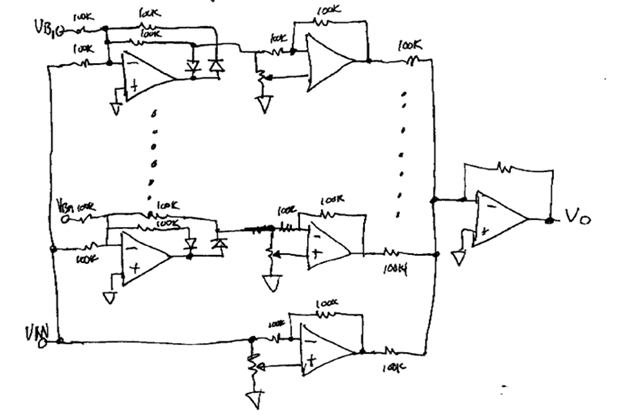## Ideal diode circuit.

### I. Basic circuit

#### Vin will be active when Vin < 0 volts and V1 will be active when Vin > 0. It should be noted that the voltages are inverted from the input to the outputs. The ideal diode circuit is equivalent to a pair of diodes except the pair of diodes does not invert the voltages. Real diodes would also have a fixed voltage drop. The ideal diode circuit, thanks to the opamp and the magic of negative feedback, eliminates this voltage drop. This is what makes the circuit ideal.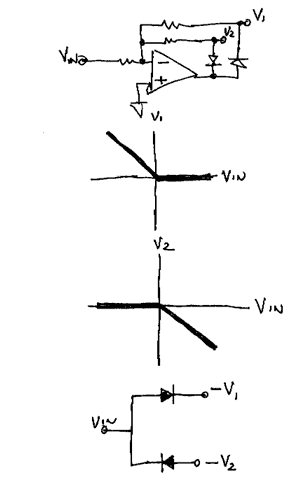### II. Full wave rectifier

#### The most common application of the ideal diode is the full wave rectifier. It works by summing one of the ideal diode outputs with the input.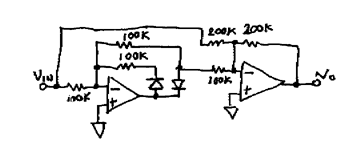### III. Piecewise linear functions

#### More basic functions are possible. As shown the gain can be made to reverse at Breakpoint. Very complex functions can be generated.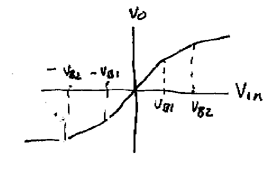#### The basic circuit is shown below. Every Breakpoint requires an ideal diode circuit. Complex functions with lots of breakpoints will require a lot of ideal diode circuits.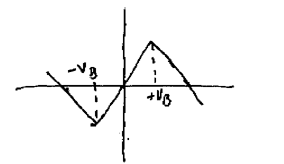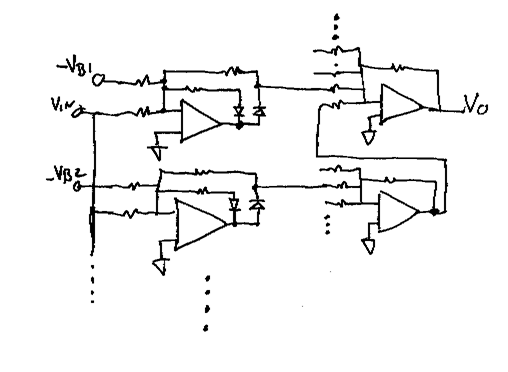### IV. Practical Examples - Voltage Splitter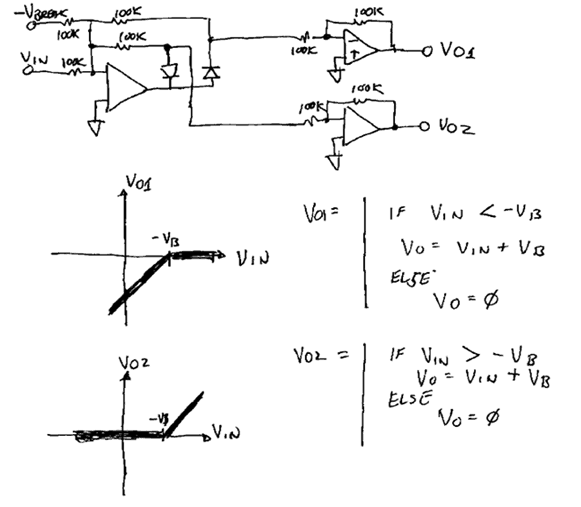### V. Practical Examples - Voltage Scanner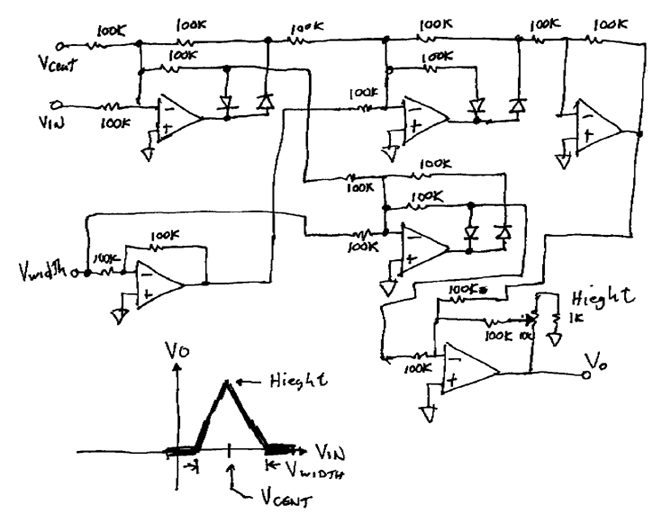### VI. Practical Examples - Waveform Shaper